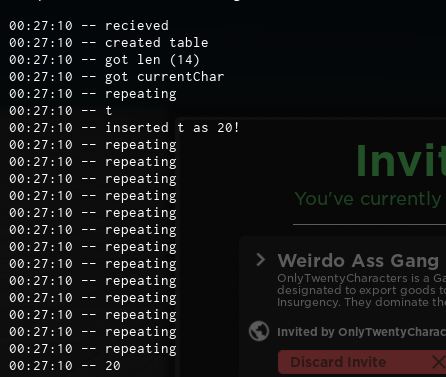# Code block gets somehow only executed once?

Hey devs!
So what I am trying to do is generating a Code out of a string. My plan was using the Alphabet for that and for loops. The number generated as the letter is the index, the problem is, it is only inserting the first letter in my Table. In this example it would be 20 since t is the 20th letter in my table. Does anybody know why?

And sorry for my bad english, explaining in a foreign language is hard ;=)

``````local alphabet = {"a","b","c","d","e","f","g","h","i","j","k","l","m","n","o","p","q","r","s","t","u","v","w","x","y","z","A","B","C","D","E","F","G","H","I","J","K","L","M","N","O","P","Q","R","S","T","U","V","W","X","Y","Z"}

function generateID(gangName)
print("recieved")
local finalProduct = {}
print("created table")
local len = string.len(gangName)
print("got len ("..len..")")
local currentChar = 0
print("got currentChar")
repeat
print("repeating")
wait()
for i, v in pairs(alphabet) do
if string.sub(gangName, currentChar, currentChar + 1) == v then
print(string.sub(gangName, currentChar, currentChar + 1))
table.insert(finalProduct, #finalProduct, i)
print("inserted "..v.." as "..i.."!")
end
end
currentChar = currentChar + 1
until currentChar == len
for i, v in pairs(finalProduct) do
print(v)
end
return finalProduct
end

local result = generateID("tankkillera10c")
print(result)
``````
1 Like

It looks like you need to put the `currentChar` variable into the `for i, v in pairs()` loop.

It only adds a character AFTER the loop finishes, so because it doesn’t add 1 to ‘currentChar’ until the loop finishes, the counter never goes up, so it only prints the first character.

1 Like
``````local alphabet = {"a","b","c","d","e","f","g","h","i","j","k","l","m","n","o","p","q","r","s","t","u","v","w","x","y","z","A","B","C","D","E","F","G","H","I","J","K","L","M","N","O","P","Q","R","S","T","U","V","W","X","Y","Z"}

function generateID(gangName)
print("recieved")
local finalProduct = {}
print("created table")
local len = string.len(gangName)
print("got len ("..len..")")
local currentChar = 0
print("got currentChar")
repeat
print("repeating")
wait()
for i, v in pairs(alphabet) do
if string.sub(gangName, currentChar, currentChar + 1) == v then
print(string.sub(gangName, currentChar, currentChar + 1))
table.insert(finalProduct, #finalProduct, i)
print("inserted "..v.." as "..i.."!")
currentChar = currentChar + 1
end
end
until currentChar == len
for i, v in pairs(finalProduct) do
print(v)
end
return finalProduct
end

local result = generateID("tankkillera10c")
print(result)
``````

Here’s the fixed code. Hope this helps1 Like

This spams the console with repeating cuz it doesnt seem to stop.
I was putting it outside of the loop since its in a repeat until. I

This is what it now looks.This is what it looked before.

Came a whole step further.

``````local alphabet = {"a","b","c","d","e","f","g","h","i","j","k","l","m","n","o","p","q","r","s","t","u","v","w","x","y","z","A","B","C","D","E","F","G","H","I","J","K","L","M","N","O","P","Q","R","S","T","U","V","W","X","Y","Z"}

function generateID(gangName)
print("recieved")
local finalProduct = {}
print("created table")
local len = string.len(gangName)
print("got len ("..len..")")
local currentChar = 0
print("got currentChar")
repeat
print("repeating")
wait()
for i, v in pairs(alphabet) do

if string.sub(gangName, currentChar, currentChar) == v then
print(string.sub(gangName, currentChar, currentChar))
table.insert(finalProduct, #finalProduct, i)
print("inserted "..v.." as "..i.."!")
end
end
currentChar = currentChar + 1
until currentChar == len
for i, v in pairs(finalProduct) do
print(v)
end
return finalProduct
end

local result = generateID("Velexty")
``````

It seems the issue was my string.sub.

1 Like

Another bug however, it doesnt insert in the table.

This is the output.

1 Like

Quick question: How do you want numbers to be added to the code/message?

This slightly modified code that uses string.find seems to work:

``````local alphabet = "abcdefghijklmnopqrstuvwxyzABCDEFGHIJKLMNOPQRSTUVWXYZ"

function generateID(gangName)
print("recieved")
local finalProduct = {}
print("created table")
local length = string.len(gangName)
print("got len ("..length..")")

local i = 1
while i <= length do
local char = string.sub(gangName,i,i)
local first, last = string.find(alphabet, char)
table.insert(finalProduct, first)

i += 1
end

for i, v in pairs(finalProduct) do
print(v)
end

return finalProduct
end

local result = generateID("tankkillera10c")
print(result)
``````
3 Likes

It shoud look like this at the end
{20,4,5,30,2,4}
It is important to be a table, else I can’t encode it.

1 Like